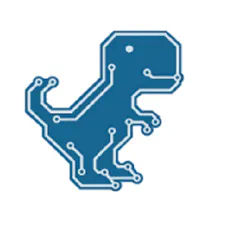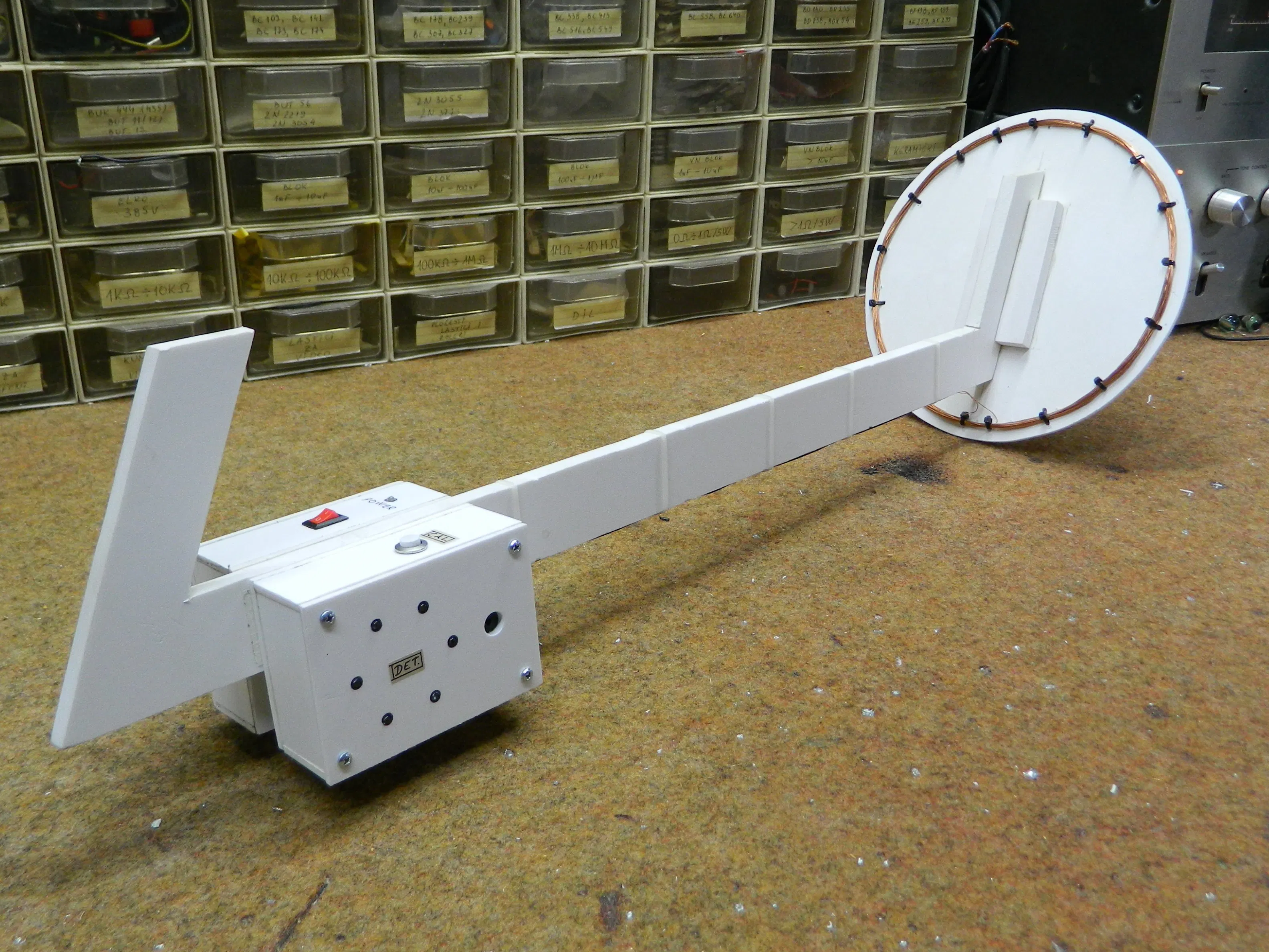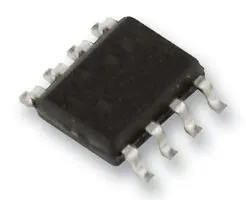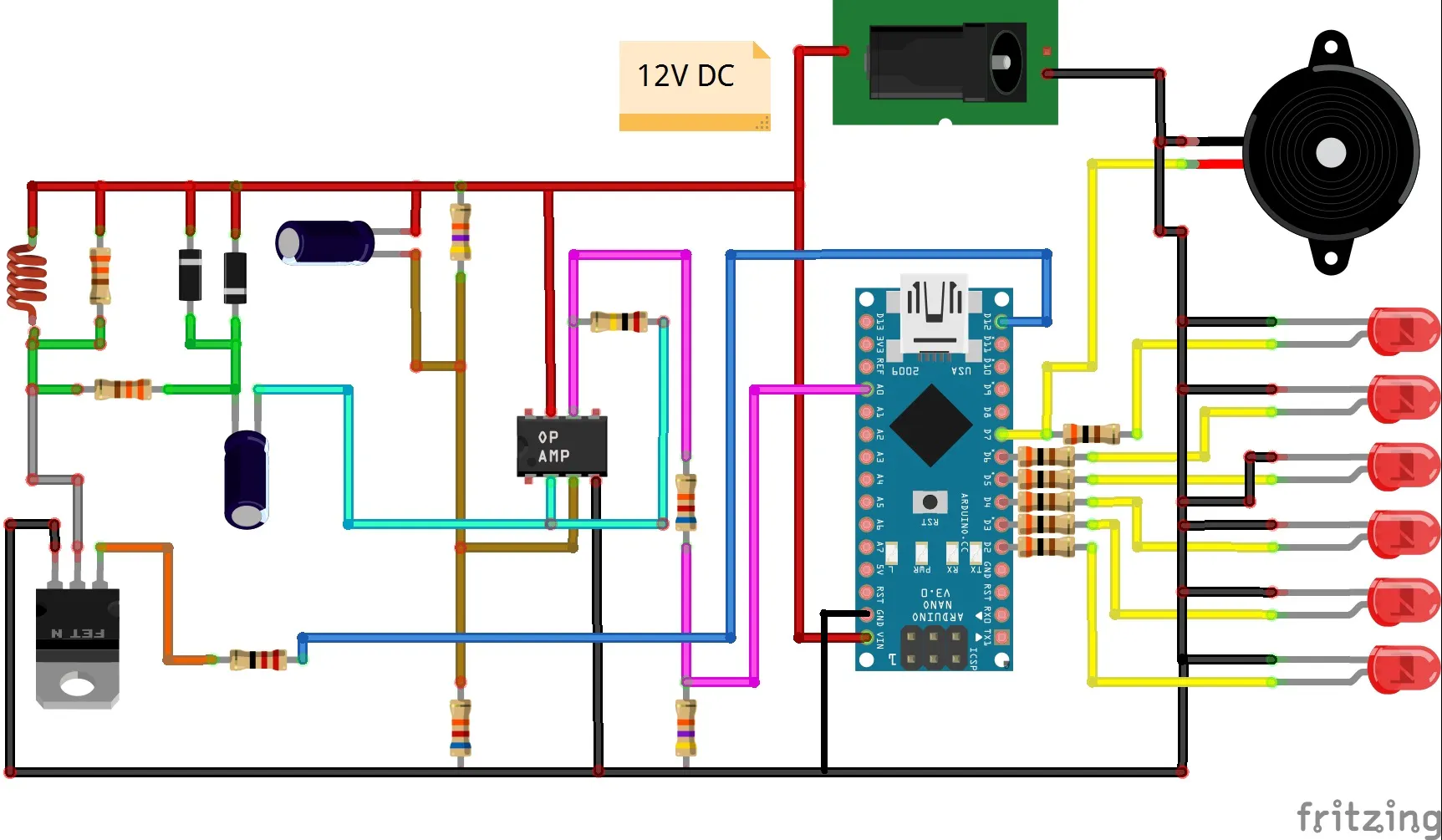# DIY Simple Sensitive Metal Detector

Pulse induction Metal Detector. It can detect a metal coin at a distance of 15 cm and a larger metal object at a distance of 40 cm and more.

IntermediateFull instructions provided19,926## Things used in this project

### Hardware componentsArduino Nano R3
×1Operational Amplifier, Op Amp + Comparator + Reference
×1
 IRF840 Poweer Mosfet
×1LED (generic)
×6
 Resistors, capacitors, diodes
×1

### Software apps and online servicesArduino IDE

### Hand tools and fabrication machinesSoldering iron (generic)

## Schematics

### Schematic## Code

### Arduino Code

C/C++
```// PI metal detector for arduino version_18_min (C) alex --- 1967 2015
int ss0 = 0;
int ss1 = 0;
int ss2 = 0;
long c0 = 0;
long c1 = 0;
long c2 = 0;
byte i = 0;
int sss0 = 0;
int sss1 = 0;
int sss2 = 0;
int s0 = 0;
int s1 = 0;
int s2 = 0;

void setup ()
{
DDRB = 0xFF; // port B - all out
DDRD = 0xFF; // port D - all out

for (i = 0; i <255; i ++) // calibration / calibration
{
PORTB = B11111111; // translate the pins of port B to 1, thus opening. key (turn on)
delayMicroseconds (200); // wait 200 microseconds
PORTB = 0; //
delayMicroseconds (20);
c0 = c0 + s0;
c1 = c1 + s1;
c2 = c2 + s2;

delay (3);
}
c0 = c0 / 255;
c0 = c0 - 5;
c1 = c1 / 255;
c1 = c1 - 5;
c2 = c2 / 255;
c2 = c2 - 5;

}

void loop ()
{
PORTB = B11111111; // translate the pins of port B to 1, thus opening. key (turn on)
delayMicroseconds (200); // wait 200 microseconds
PORTB = 0; // close the key by interrupting the current in the search coil (turn off - interrupt circut in search coil)
delayMicroseconds (20);
ss0 = s0 - c0;

if (ss0 <0)
{
sss0 = 1;
}
ss0 = ss0 / 16;
PORTD = ss0; // send to the indicator (send to LEDs)
delay (1);

ss1 = s1 - c1;
if (ss1 <0)
{
sss1 = 1;
}
ss1 = ss1 / 16;
PORTD = ss1; // send to the indicator (send to LEDs)
delay (1);

ss2 = s2 - c2;
if (ss2 <0)
{
sss2 = 1;
}
ss2 = ss2 / 16;
PORTD = ss2; // send to the indicator (send to LEDs)
delay (1);

if (sss0 + sss1 + sss2> 2)
{
digitalWrite (7, HIGH);
digitalWrite (6, HIGH);
digitalWrite (5, HIGH);
digitalWrite (4, HIGH);
digitalWrite (3, HIGH);
digitalWrite (2, HIGH);
digitalWrite (1, HIGH);
digitalWrite (0, HIGH);
delay (1);
sss0 = 0;
sss1 = 0;
sss2 = 0;
}
}
```

## Credits

### Mirko Pavleski

22 projects • 556 followers